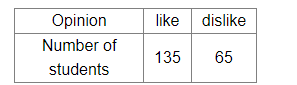# To know the opinion of the students about the subject Sanskrit, a survey of 200 students was conducted.Question:

To know the opinion of the students about the subject Sanskrit, a survey of 200 students was conducted. The data is recorded as under.What is the probability that a student chosen at random does not like it?

(a) $\frac{13}{27}$

(b) $\frac{27}{40}$

(c) $\frac{13}{40}$

(d) $\frac{27}{13}$

Solution:

Total number of students surveyed = 200

Number of students who does not like the subject Sanskrit = 65

$\therefore \mathrm{P}($ Student chosen at random does not like the subject Sanskrit) $)=\frac{\text { Number of students who does not like the subject Sanskrit }}{\text { Total number of students surveyed }}=\frac{65}{200}=\frac{13}{40}$

Hence, the correct answer is option (c).